# Stoichiometry Introduction to Stoichiometry I can define stoichiometry

• Slides: 69StoichiometryIntroduction to Stoichiometry�I can define stoichiometry. �I can identify the number of moles required in a reaction based on the coefficients. �I can determine how many moles of product will be produced based on the molar ratios found in an equation.�Note Expectations: Cell phones and electronics are not in use. You are taking the notes. You are helping the people at your table to answer the questions. You are prepared to answer the questions.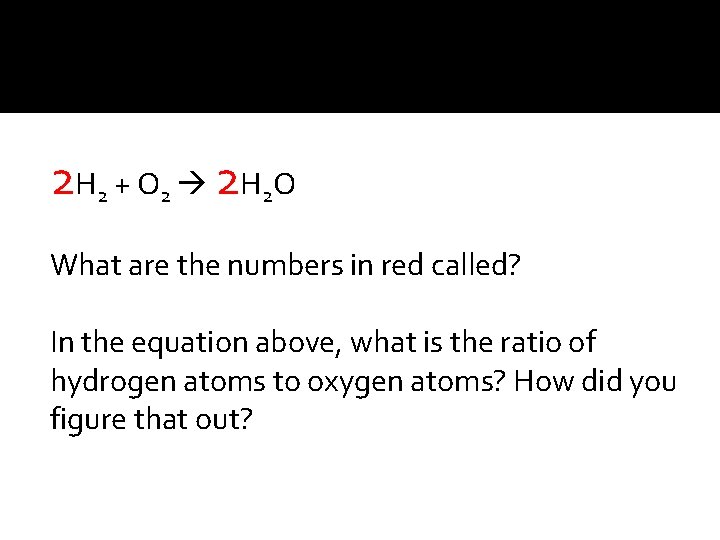2 H 2 + O 2 2 H 2 O What are the numbers in red called? In the equation above, what is the ratio of hydrogen atoms to oxygen atoms? How did you figure that out?2 H 2 + O 2 2 H 2 O If you have 6 hydrogen atoms, how many oxygen atoms do you need to react with all of the hydrogen atoms? Why? If you have 8 hydrogen atoms and 4 oxygen atoms, how many water molecules would you make? How did you figure out your answer?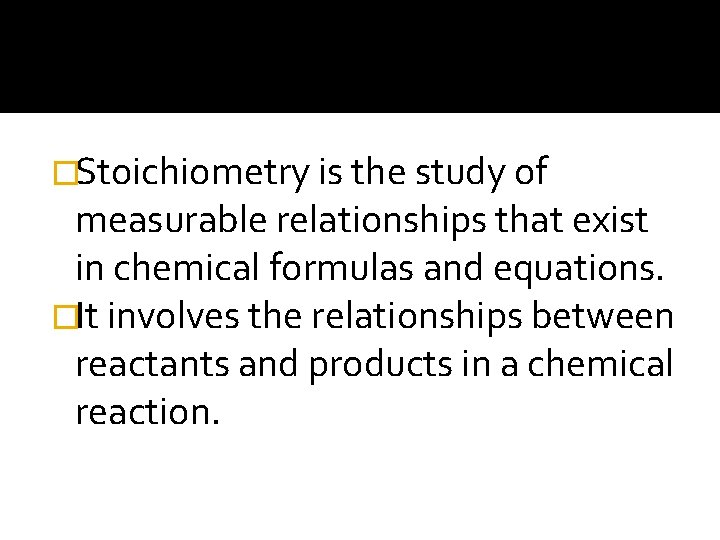�Stoichiometry is the study of measurable relationships that exist in chemical formulas and equations. �It involves the relationships between reactants and products in a chemical reaction.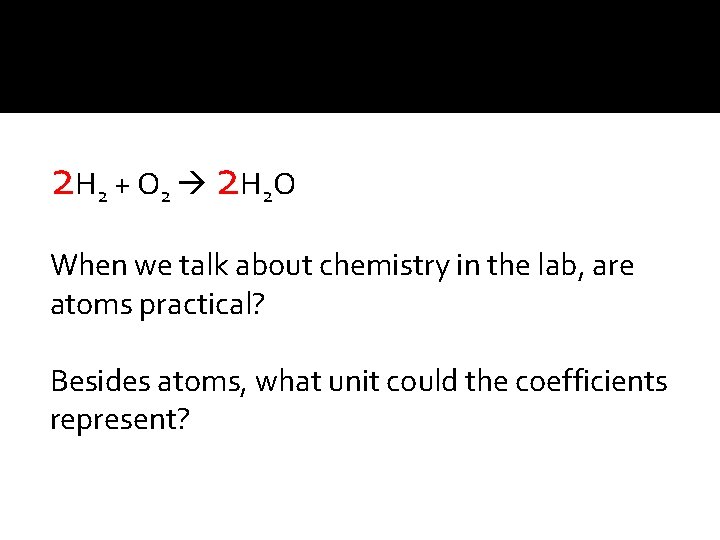2 H 2 + O 2 2 H 2 O When we talk about chemistry in the lab, are atoms practical? Besides atoms, what unit could the coefficients represent?2 H 2 + O 2 2 H 2 O If you have 8 moles of hydrogen, how many moles of oxygen do you need to react with all of the hydrogen atoms? Why?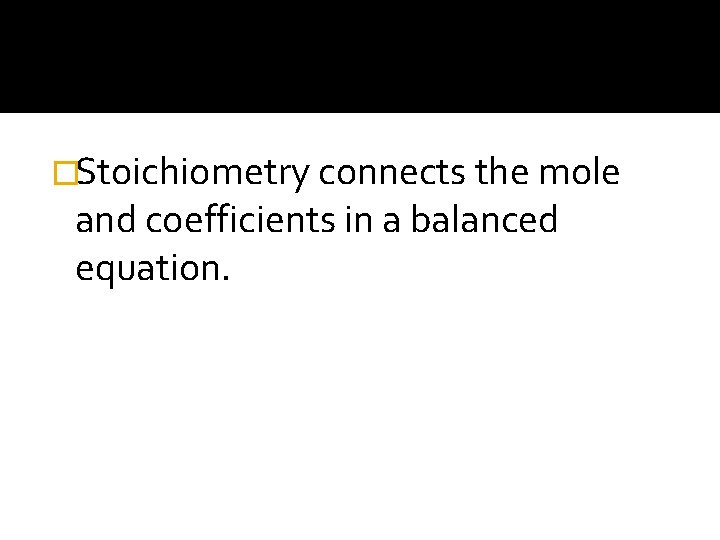�Stoichiometry connects the mole and coefficients in a balanced equation.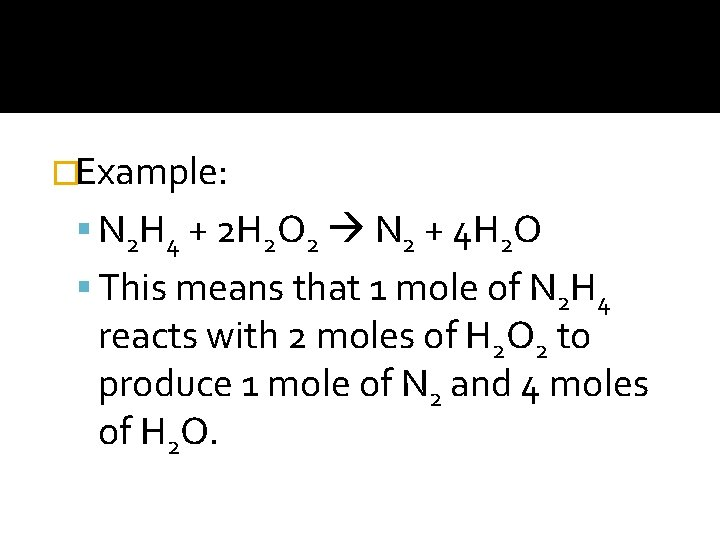�Example: N 2 H 4 + 2 H 2 O 2 N 2 + 4 H 2 O This means that 1 mole of N 2 H 4 reacts with 2 moles of H 2 O 2 to produce 1 mole of N 2 and 4 moles of H 2 O.�There is now a relationship between the coefficients and moles. �Molar ratios allow you to determine the number of moles of any substance in the reaction.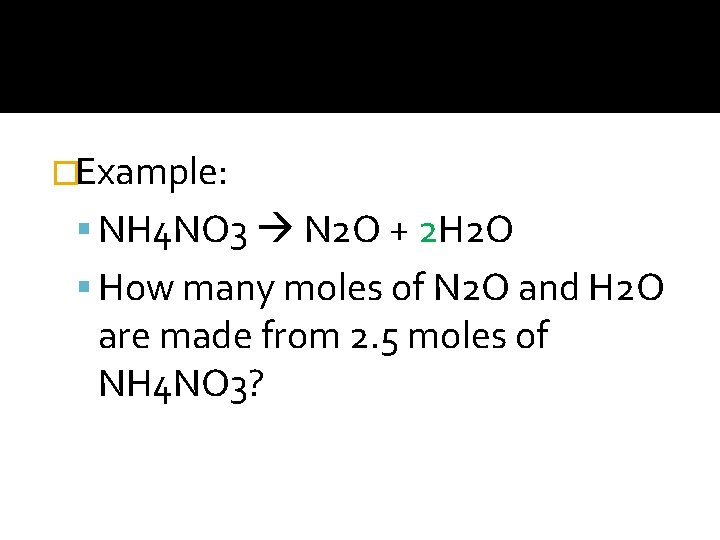�Example: NH 4 NO 3 N 2 O + 2 H 2 O How many moles of N 2 O and H 2 O are made from 2. 5 moles of NH 4 NO 3?�You can convert from moles of one substance to moles of another substance using mole ratios.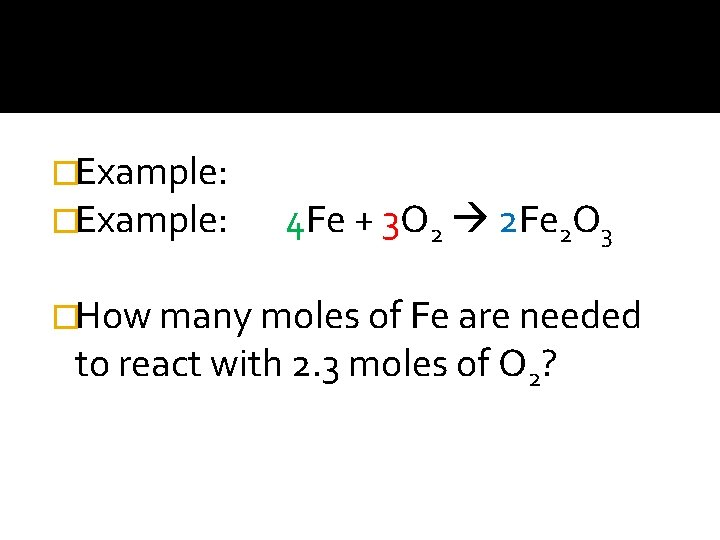�Example: 4 Fe + 3 O 2 2 Fe 2 O 3 �How many moles of Fe are needed to react with 2. 3 moles of O 2?�Balanced equations follow the law of conservation of matter. �The mass of the products equals the mass of the reactants.�What is stoichiometry? �Stoichiometry connects ______ and _________. �What is a molar ratio? �How does the mass of the products in a reaction compare to the mass of the reactants? Why?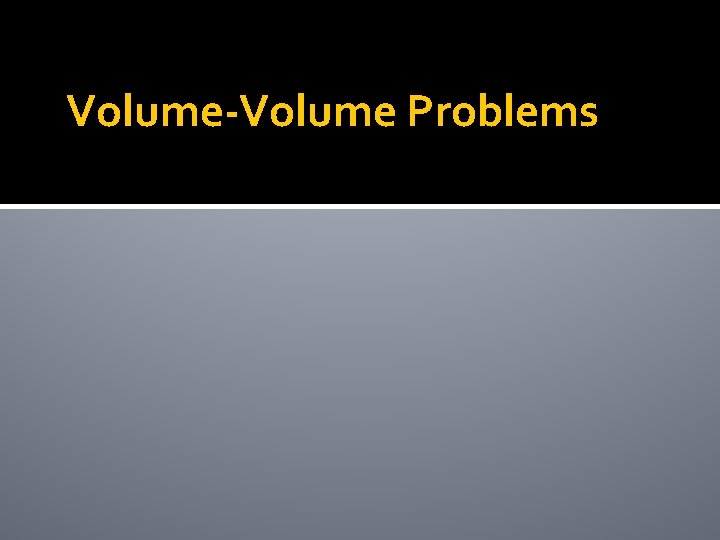Volume-Volume Problems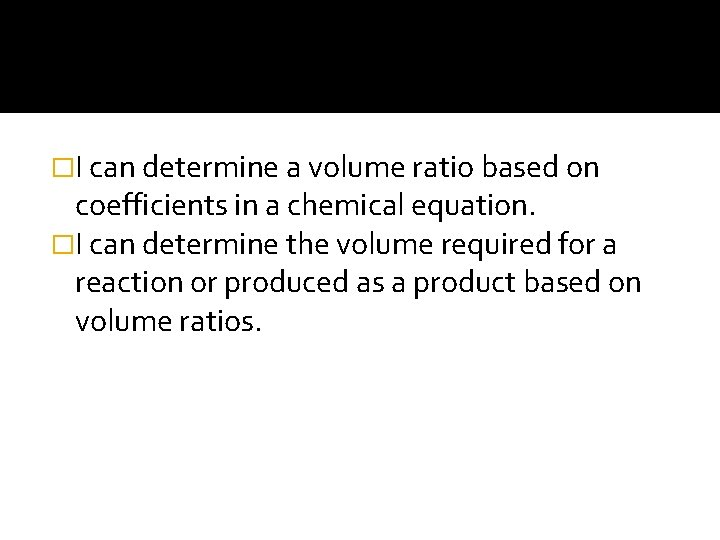�I can determine a volume ratio based on coefficients in a chemical equation. �I can determine the volume required for a reaction or produced as a product based on volume ratios.�Note Expectations: Cell phones and electronics are not in use. You are taking the notes. You are helping the people at your table to answer the questions. You are prepared to answer the questions.�What is the volume of 1 mole of gas at STP? �Is this a constant or does it change based on the type of gas?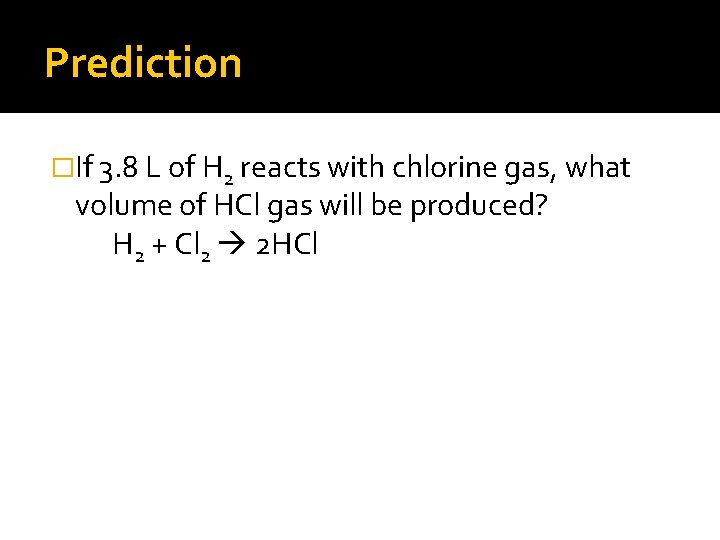Prediction �If 3. 8 L of H 2 reacts with chlorine gas, what volume of HCl gas will be produced? H 2 + Cl 2 2 HCl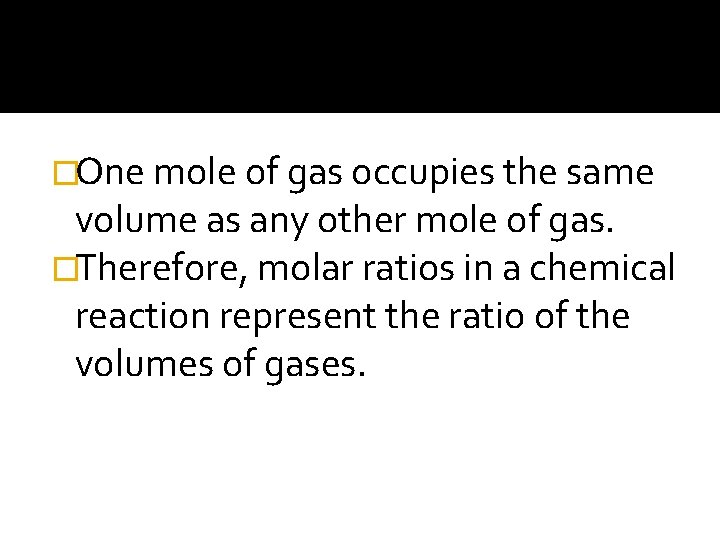�One mole of gas occupies the same volume as any other mole of gas. �Therefore, molar ratios in a chemical reaction represent the ratio of the volumes of gases.�Volume-volume problems give you a volume and ask you to find the volume of an unknown. �You will use the volume ratio from the equation to solve these problems.�What unit is used to measure volume?�The volume ratio is the same as a molar ratio, except it uses liters.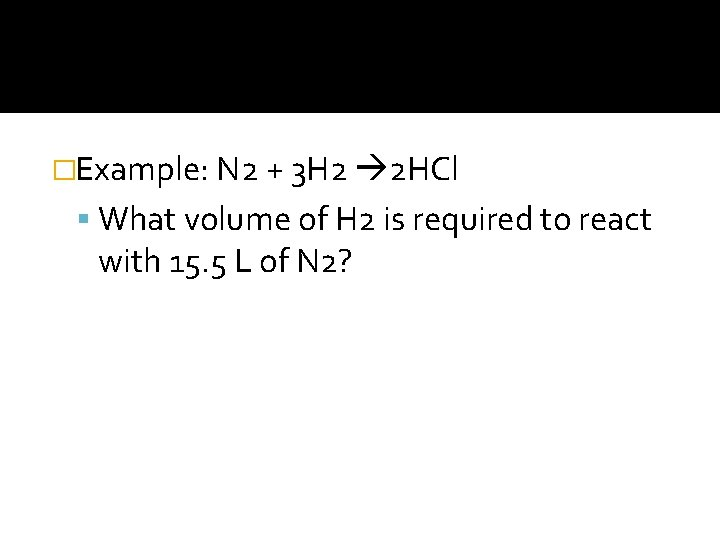�Example: N 2 + 3 H 2 2 HCl What volume of H 2 is required to react with 15. 5 L of N 2?�Example: If. 38 L of H 2 reacts with chlorine gas, what volume of HCl gas will be produced? H 2 + Cl 2 2 HCl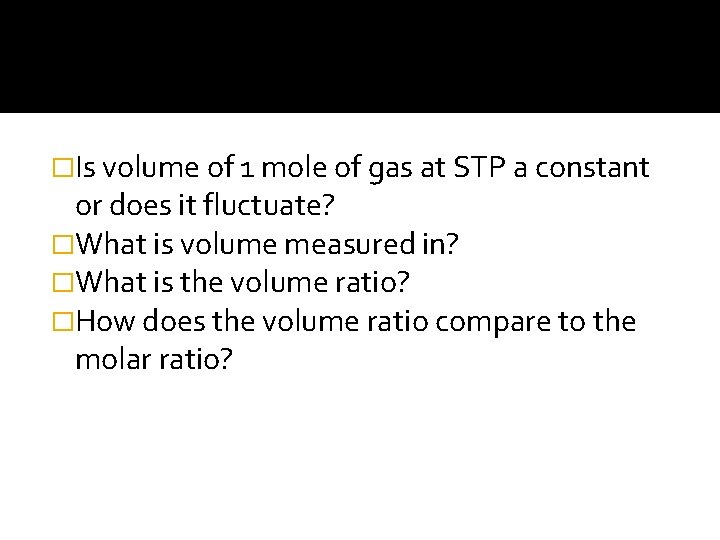�Is volume of 1 mole of gas at STP a constant or does it fluctuate? �What is volume measured in? �What is the volume ratio? �How does the volume ratio compare to the molar ratio?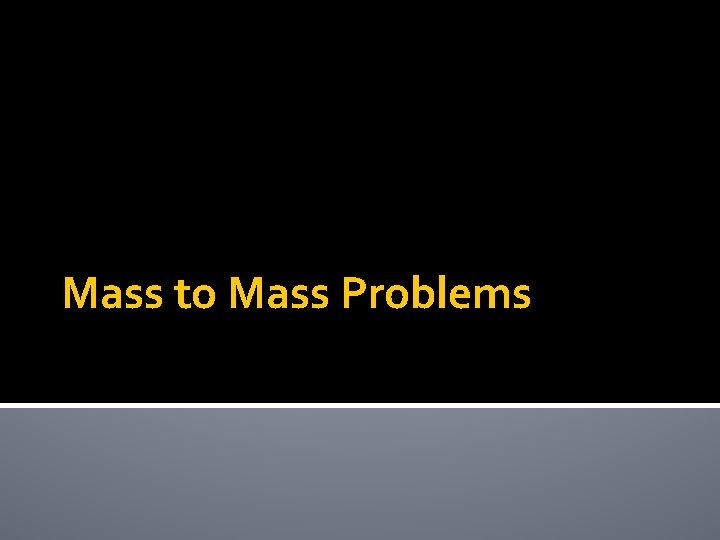Mass to Mass Problems�I can determine the mass required or produced during a chemical reaction based on the molar ratios found in a chemical equation.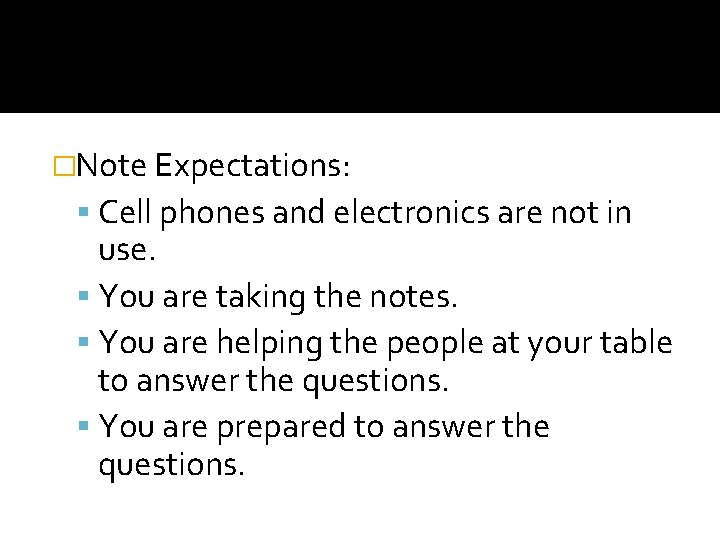�Note Expectations: Cell phones and electronics are not in use. You are taking the notes. You are helping the people at your table to answer the questions. You are prepared to answer the questions.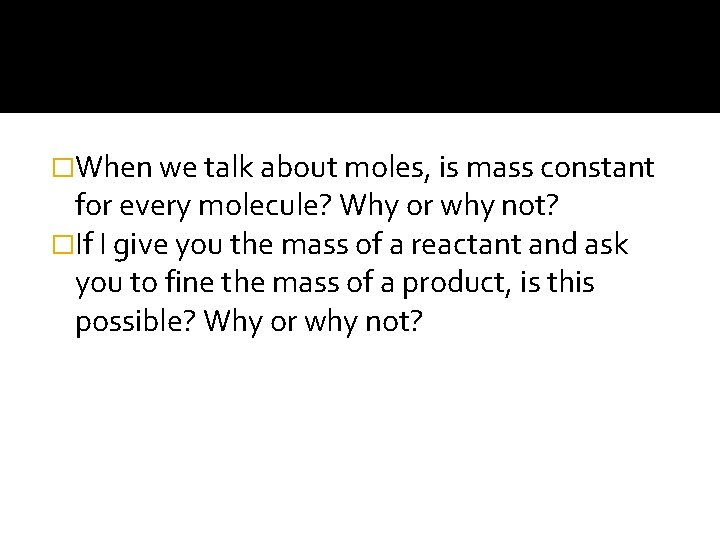�When we talk about moles, is mass constant for every molecule? Why or why not? �If I give you the mass of a reactant and ask you to fine the mass of a product, is this possible? Why or why not?�Since a chemical equation is related to moles, you can now do mole conversions using the information in an equation.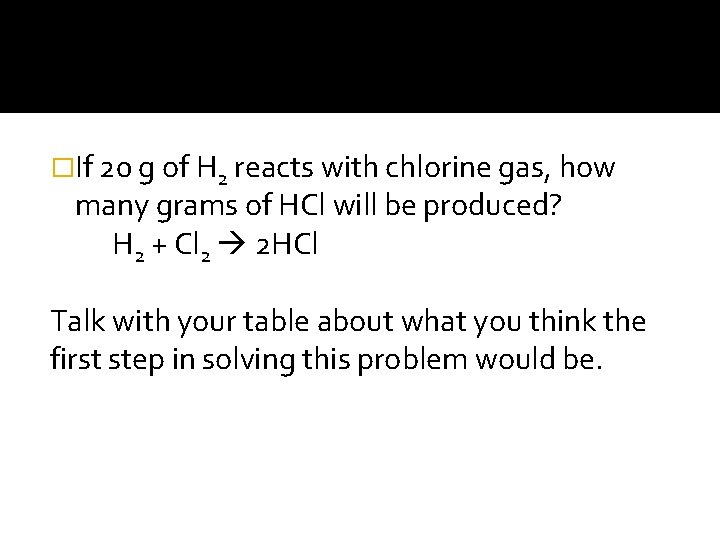�If 20 g of H 2 reacts with chlorine gas, how many grams of HCl will be produced? H 2 + Cl 2 2 HCl Talk with your table about what you think the first step in solving this problem would be.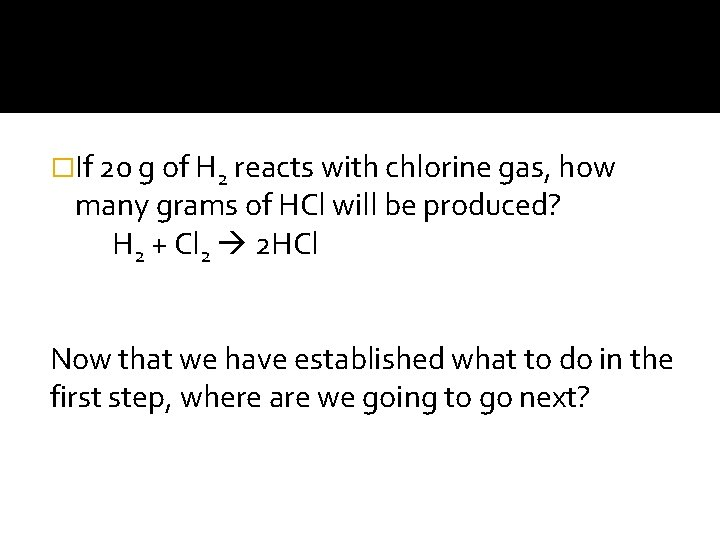�If 20 g of H 2 reacts with chlorine gas, how many grams of HCl will be produced? H 2 + Cl 2 2 HCl Now that we have established what to do in the first step, where are we going to go next?�If 20 g of H 2 reacts with chlorine gas, how many grams of HCl will be produced? H 2 + Cl 2 2 HCl What will the final step in this problem be?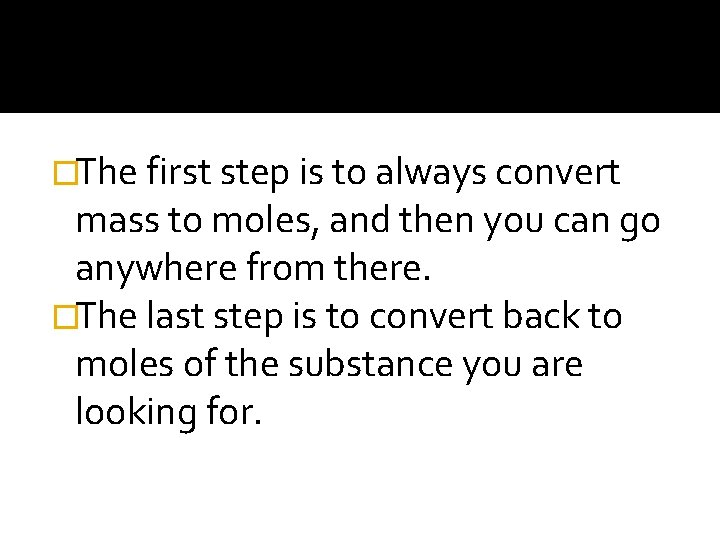�The first step is to always convert mass to moles, and then you can go anywhere from there. �The last step is to convert back to moles of the substance you are looking for.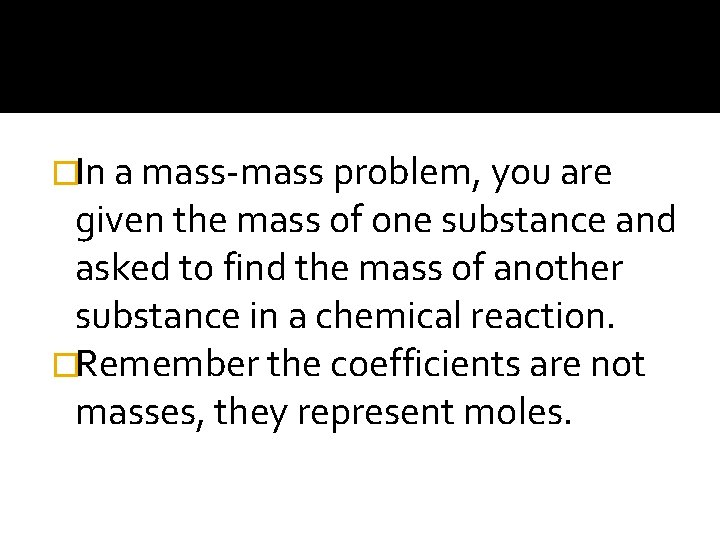�In a mass-mass problem, you are given the mass of one substance and asked to find the mass of another substance in a chemical reaction. �Remember the coefficients are not masses, they represent moles.�What mass of water is produced from 1. 5 grams of glucose (C 6 H 12 O 6)? C 6 H 12 O 6 + 6 O 2 6 CO 2 + 6 H 2 O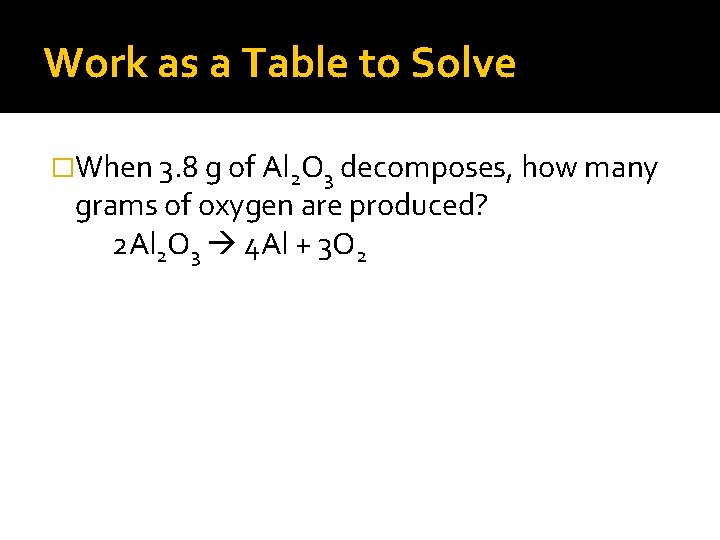Work as a Table to Solve �When 3. 8 g of Al 2 O 3 decomposes, how many grams of oxygen are produced? 2 Al 2 O 3 4 Al + 3 O 2�Is mass a constant for every molecule? Why or why not? �In a mass-mass problem what are you looking for?Limiting Reactants�I can define a limiting reactant. �I can determine the limiting reactant for a reaction based on the amount of reactants used in a reaction.�Note Expectations: Cell phones and electronics are not in use. You are taking the notes. You are helping the people at your table to answer the questions. You are prepared to answer the questions.�Can chemical reactions go on and on forever? Why or why not?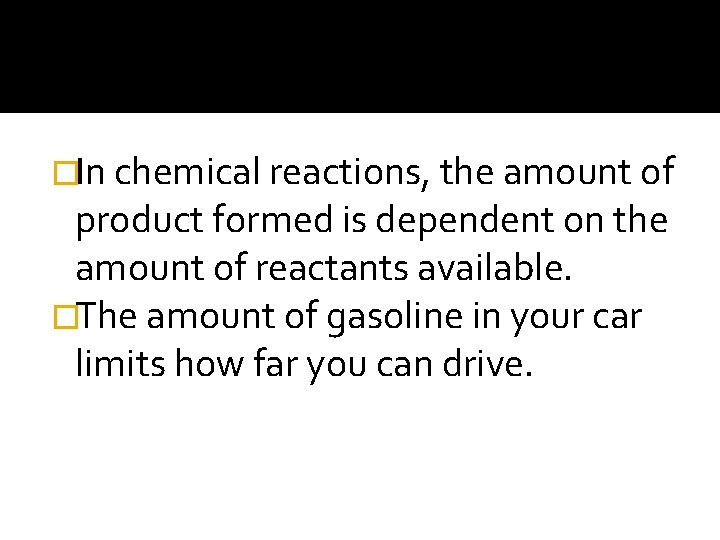�In chemical reactions, the amount of product formed is dependent on the amount of reactants available. �The amount of gasoline in your car limits how far you can drive.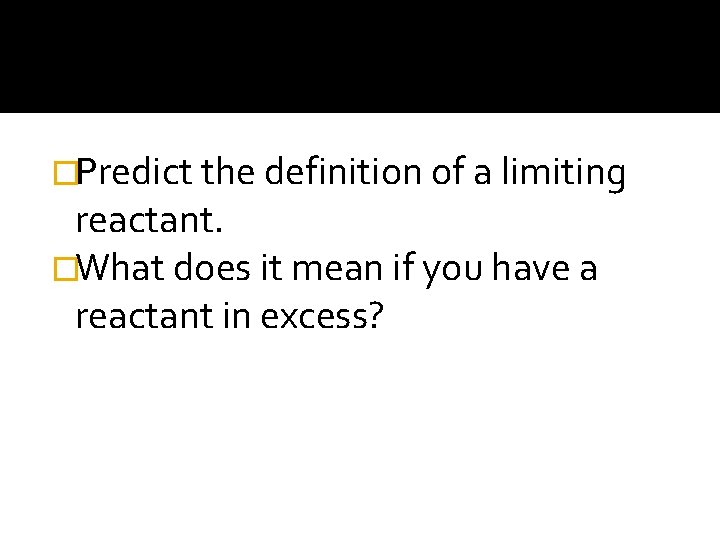�Predict the definition of a limiting reactant. �What does it mean if you have a reactant in excess?�Often, in a chemical reaction, there is a reactant that limits the amount of product that can be formed. �A limiting reactant is one that limits the amount of product formed in a reaction.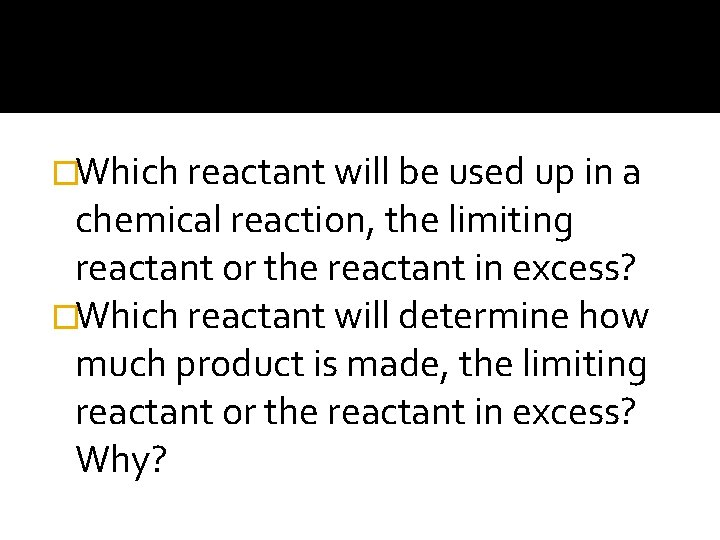�Which reactant will be used up in a chemical reaction, the limiting reactant or the reactant in excess? �Which reactant will determine how much product is made, the limiting reactant or the reactant in excess? Why?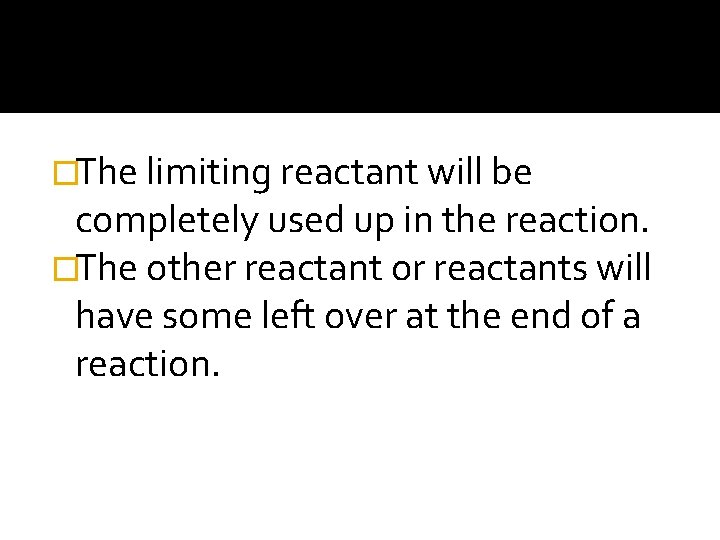�The limiting reactant will be completely used up in the reaction. �The other reactant or reactants will have some left over at the end of a reaction.�The leftover reactant is said to be in excess. �The amount of product is always determined by the limiting reactant.If you have 3. 5 g of Cu and 6. 0 g of Ag. NO 3, which reactant is the limiting reactant in the production of silver? Cu + 2 Ag. NO 3 Cu(NO 3)2 + 2 Ag Predict how you would solve this problem. What are you going to look for?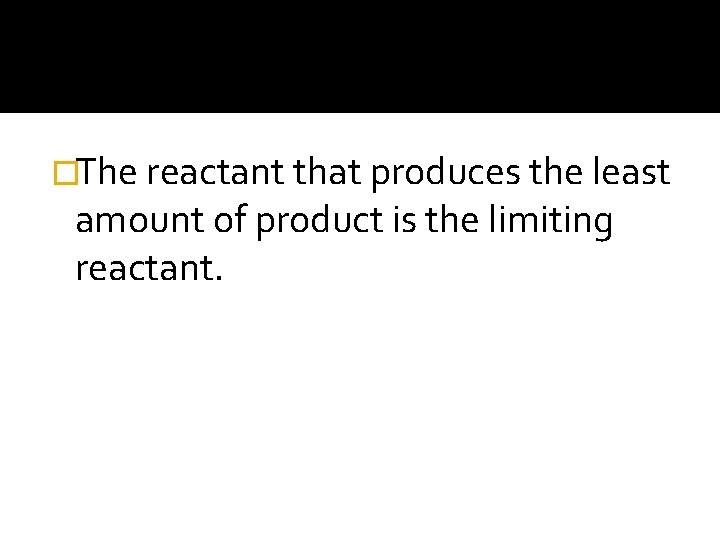�The reactant that produces the least amount of product is the limiting reactant.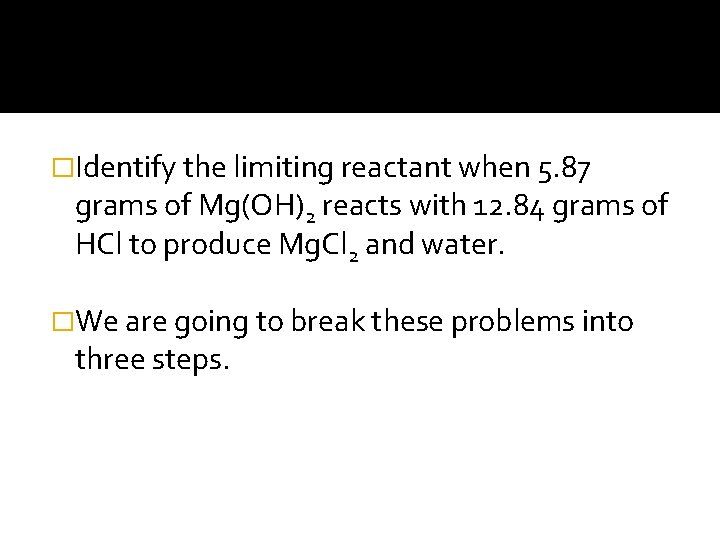�Identify the limiting reactant when 5. 87 grams of Mg(OH)2 reacts with 12. 84 grams of HCl to produce Mg. Cl 2 and water. �We are going to break these problems into three steps.Step 1 How much water is produced if you start with 5. 87 grams of Mg(OH)2? Mg(OH)2 + 2 HCl Mg. Cl 2 + 2 H 2 OStep 2 �How much water is produced if you start with 12. 84 grams of HCl? Mg(OH)2 + 2 HCl Mg. Cl 2 + 2 H 2 OStep 3 �Circle the limiting reactant: Mg(OH)2 or HCl.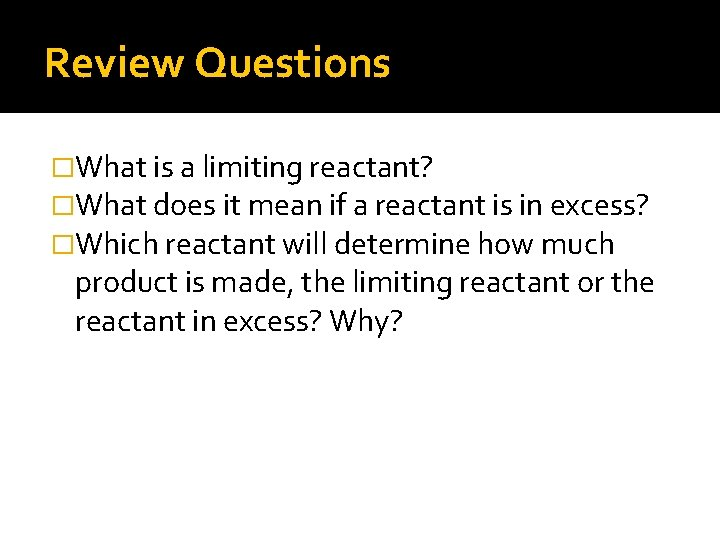Review Questions �What is a limiting reactant? �What does it mean if a reactant is in excess? �Which reactant will determine how much product is made, the limiting reactant or the reactant in excess? Why?Percent Yield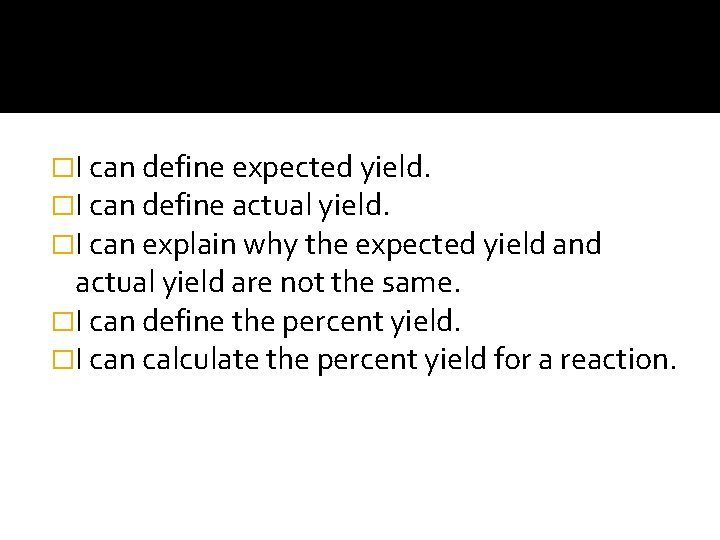�I can define expected yield. �I can define actual yield. �I can explain why the expected yield and actual yield are not the same. �I can define the percent yield. �I can calculate the percent yield for a reaction.�The amount of product that is produced in a reaction is usually less than the predicted amounts. �The amount of product that should be produced based on calculations is the expected yield.�The amount of product really obtained is the actual yield. �Product can be lost for several reason. �The reactants may not react.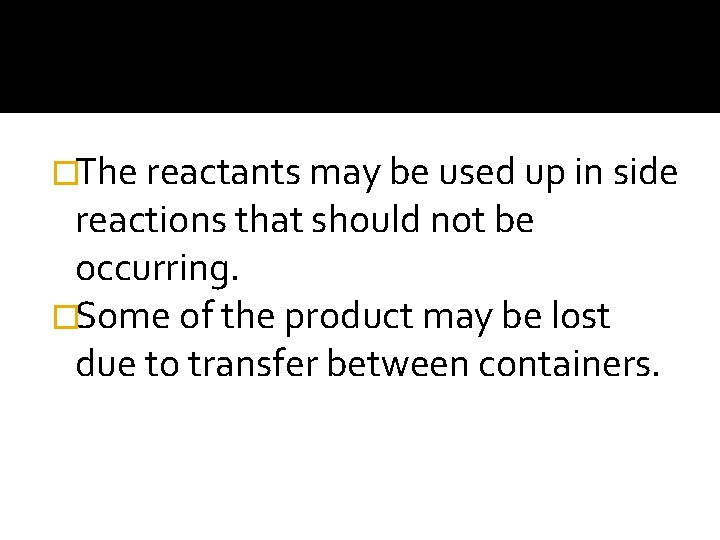�The reactants may be used up in side reactions that should not be occurring. �Some of the product may be lost due to transfer between containers.�It is useful to determine what percent of the expected yield was actually obtained. �This percentage is called percent yield.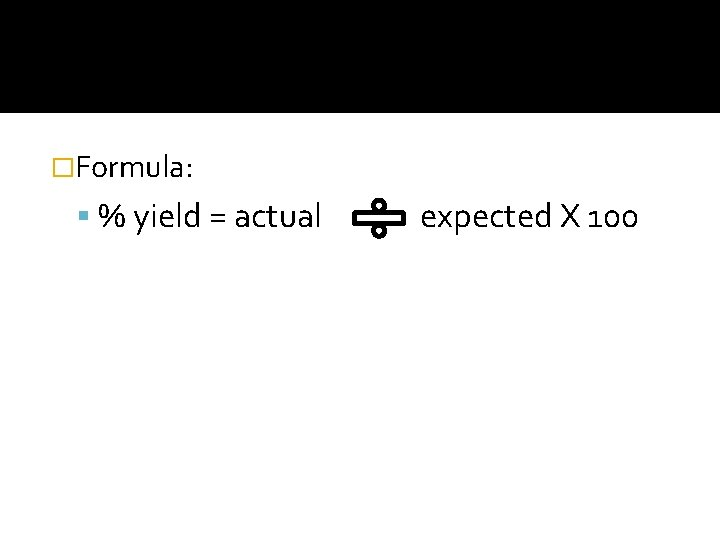�Formula: % yield = actual expected X 100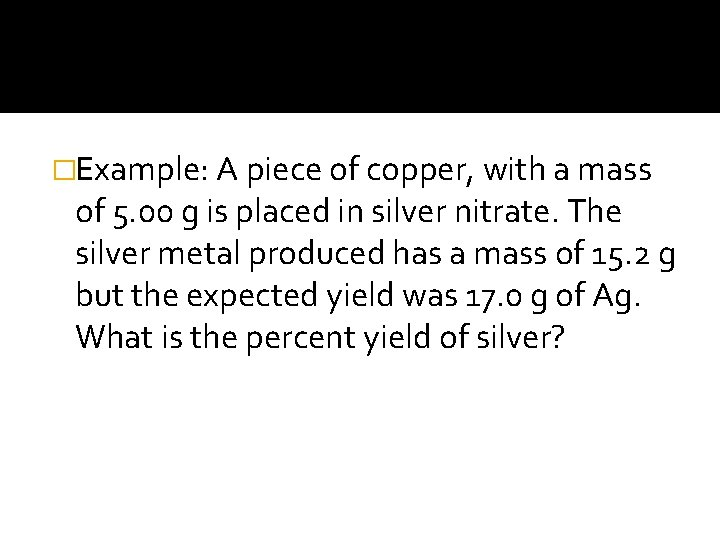�Example: A piece of copper, with a mass of 5. 00 g is placed in silver nitrate. The silver metal produced has a mass of 15. 2 g but the expected yield was 17. 0 g of Ag. What is the percent yield of silver?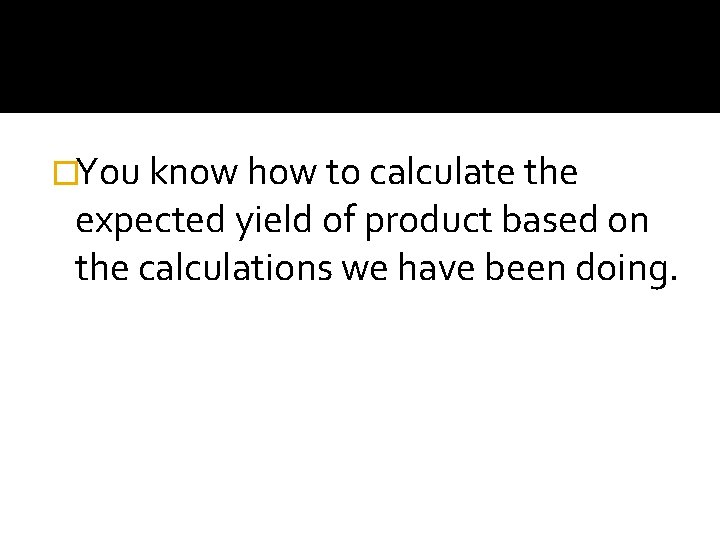�You know how to calculate the expected yield of product based on the calculations we have been doing.�Example: Determine the percent yield for the reaction between 2. 8 g Al(NO 3)3 and excess Na. OH if 0. 966 g AL(OH)3 is recovered. Al(NO 3)3 + Na. OH Al(OH)3 + 3 Na. NO 3 First calculate how much Al(OH)3 should have been produced, then calculate the percent yield.